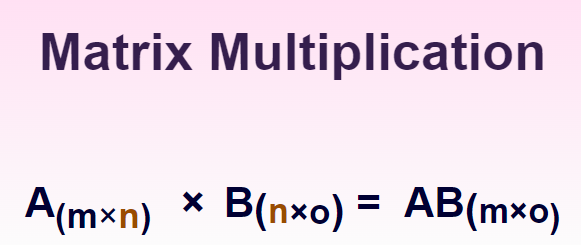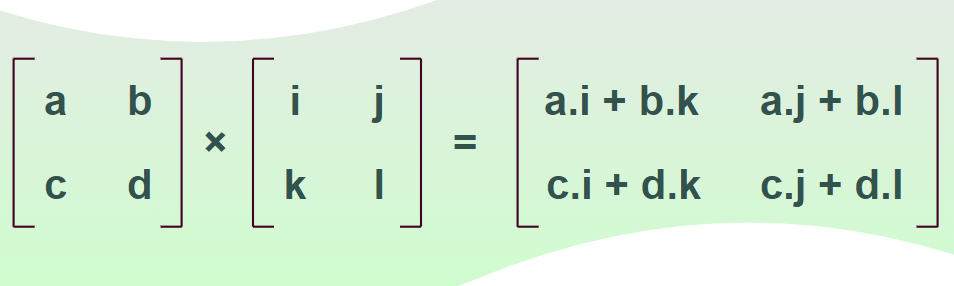# Matrix Multiplication

Matrix Multiplication is a binary operation performed on two matrices to get a new matrix called the product matrix. Suppose we take two matrices A and B such that the number of columns in the first matrix is equal to the number of rows in the second matrix then we can multiply these two matrices to get a new matrix of the same order that is called the multiplication of the two matrices A and B. Thus, it is clear that not any two matrices can be multiplied and we can multiply only those matrices that follow a specific condition.

French mathematician Jacques Philippe Marie Binet was the first to perform matrix multiplication in 1812. Now let’s learn more about matrix multiplication its properties and others in detail.

## What is Matrix Multiplication?

Matrix multiplication is the mathematical operation that is performed on two matrices which when multiplied gives a singular matrix. As we can perform multiplication on any two numbers we can not perform multiplication on any two matrices. We have to follow specific rules to perform matrix multiplication and the matrices obeying a certain relation are the matrices that can be multiplied.

The multiplication of any two matrices gives a single matrix, and it is possible only if the number of columns in the 1st matrix is equal to the number of rows in the 2nd matrix. If “A = [aij]m×n” and “B = [bij]n×p” are two matrices, then the product of A and B is denoted as AB, whose order is “m × p.” this condition is represented as,Usually, matrix multiplication is not commutative, i.e., AB ≠ BA, or, in simple words, the product of A and B matrices is AB and AB is not equal to BA it is even possible that AB exists but BA does not exist.

## How to Multiply Matrices?

Multiplication of the matrix follows a special rule to find the product. Suppose we have to find the multiplication of two matrices A and B where the number of columns in A is equal to the number of rows in B such that the multiplication of A and B is obtained in such a way that we multiply the first row of the first matrix with the first column of the second matrix to get the first element of the multiplied matrix. We follow the steps discussed below to find the matrix multiplication.

Step 1: Check the compatibility of the matrix by checking that the number of columns in the 1st matrix equals the number of rows in the 2nd matrix.

Step 2: Multiply the elements in the first row of the first matrix with the elements in the first column of the matrix and find the sum of all the products. Then multiply the element in the first row of the first matrix with the elements of the second column in the second matrix. Repeat this process till elements of all the positions are not obtained.

Step 3: Substitute all the elements obtained in Step 2 in their respective position to find the required product matrix.

## Rules for Matrix Multiplication

Note that the multiplication of any two matrices is possible only if both matrices are compatible. We can multiply two matrices if the number of columns in the 1st matrix is equal to the number of rows in the 2nd matrix, otherwise, the given matrices cannot be multiplied.

• Let’s take a matrix of size 2×3 and another matrix of size 3×2, then we can apply the multiplication between those matrices because the number of columns in the first matrix is similar to the number of rows in the second matrix. The order of the resultant matrix is 2×2.
• Now let’s take another matrix of size 3×3 and other matrices of size 4×2, then we cannot multiply these two matrices as the number of columns in the first matrix is not equal to the number of rows in the second matrix.

## Notation

We represent a multiplication matrix as the multiplication of two matrices A and B such that the order of A is m×p and the order of B is p×n then the order of the multiplied matrix is m×n. The

X = AB

where,

• X is the resulting matrix of m×n order
• A and B are the given matrix of order m×p and p×n

## Matrix Multiplication Formula

Let’s take two matrices A and B of order 3×3 such that A = [aij] and B = [bij]. Then the multiplication of A and B is obtained in the image such that,The resultant multiplication matrix X is represented as,

X =where,

Xxy = Ax1By1 + …..+ AxbBby = Σk= 1b AxkBky

## Algorithm for Matrix Multiplication

There are various matrix multiplication algorithms that are widely used for finding matrix multiplication and some of the most common matrix multiplication algorithms are,

• Iterative Algorithm
• Divide and Conquer Algorithm
• Sub-Cubic Algorithms
• Parallel and Distributed Algorithms

These algorithms are widely used in computer programing to find the multiplication of two matrices such that the results are efficient and take lesser memory and time. They are used to find 2×2, 3×3, and 4×4, multiplication of matrices.

We use these matrix multiplication algorithms for a variety of purposes and the method to multiply matrics is similar for any order of matrix for a particular algorithm.

## 2×2 Matrix Multiplication Formula

Let us consider two matrices A and B of order “2 × 2”. Then its multiplication is achieved using the formula.## 3×3 Matrix Multiplication Formula

Let us consider two matrices P and Q of order “3 × 3”. Now, the matrix multiplication formula of “3 × 3” matrices is,We can understand this using the example of matrix multiplication discussed below.

Example: Multiply the matrices given below.Solution:## Matrix Multiplication by Scalar

A matrix can be multiplied by a scalar value, which is called scalar multiplication, and also by a matrix, which is called matrix multiplication. Scalar multiplication is the multiplication of a matrix by a scalar value. When a matrix “A = [aij]” is multiplied by a scalar value “k,” every element of the given matrix is multiplied by the scalar value. The resultant matrix is expressed as kA, where kA = k[aij] = [kaij], for all the values of i and j.

Example:## Properties of Matrix Multiplication

The following are some important properties of matrix multiplication:

### Commutative Property

The matrix multiplication is usually not commutative i.e. the multiplication of the first matrix with the second matrix is not similar to the multiplication of the second matrix with the first.

If A and B are two matrices, then AB ≠ BA.

### Associative Property

The matrix multiplication is associative in nature. If A, B, and C are three matrices, then

A(BC) = (AB)C

This property holds true if the products A(BC) and (AB)C are defined.

### Distributive Property

Distributive property also holds true for matrix multiplication. If A, B, and C are three matrices, then by applying the distributive property, we get

• A (B + C) = AB + AC
• (B + C) A = BA + CA

This property is only true if and only if A, B, and C are compatible.

### Product with a Scalar

If A and B are two matrices and AB is defined, then the product of the matrix with the scaler is defined as,

k(AB) = (kA)B = A(Bk)

where
k is a scalar value.

### Determinant of Matrix Multiplication

If A and B are two matrices and AB is defined as the multiplication product of A and B, then the determinant of the matrix “AB” is equal to the product of the determinants of matrices A and B, i.e.,

det (AB) = det A × det B

### Transpose of Matrix Multiplication

If A and B are two matrices and AB is defined as the multiplication of the two matrices then,

(AB)T = BTAT

where
T represents the transpose of a matrix

### Multiplicative Identity Property

Matrix multiplication has an identity property that states that, if we multiply a matrix A by an Identity matrix of the same order then, it results in the same matrix.

A.I = I. A = A

where
A is an n×n matrix
I is an identity matrix of order n

### Multiplicative Property of Zero

Matrix multiplication has the property of zero which states that, If a matrix is multiplied by a zero matrix, then the resultant matrix is a zero matrix.

A.0 = 0.A = 0

where 0 is the zero matrix

Also, the product of any two non-zero matrices may result in a zero matrix, i.e.,

AB = 0

Then that doesn’t mean that A = 0 or B = 0.

## Solved Examples on Matrix Multiplication

Example 1. LetandFind A×B?

Solution:=Example 2. Letand. Find A×B?

Solution:Example 3. Let,and. Find (AB + AC)?

Solution:Now calculate (AB + AC)Example 4. Let, and A2 = pA, then find the value of p?

Solution:

Calculating, A2

A2 = A×A ==Given,

A2 = pA

Taking A2 in the equation,Now,

• 8 = 2p
• -8 = -2p

p = 4

Thus, the value of p is 4

Example 5: Find the value of 3P if.

Solution:## FAQs on Matrix Multiplication

### Q1: Define Matrix Multiplication.

In linear algebra, matrix multiplication is one of the binary operations that can be performed on matrices. The multiplication of any two matrices is possible only when the number of columns in the first matrix is equal to the number of rows in the second matrix.

### Q2: Can you Multiply Matrices of Order 2×3 and 2×2?

We know that the multiplication of any two matrices is possible only when the number of columns in the first matrix is equal to the number of rows in the second matrix.

Here, the first matrix of order 2×3 has 2 columns, while the second matrix of order 2×2 has 3 rows. Now the number of columns in the first matrix(3) is not equal to the number of rows in the second matrix(2). Hence the matrix can not be multiplied.

### Q3: How to find the Multiplication of Two Matrices?

The multiplication of any two matrices is achieved only when the number of columns in the first matrix equals the number of rows in the second matrix. That is the order of the matrices should be like Am×n and Bn×p now if we multiply the matrices A and B we get,

A×B = [AB]m×p

### Q4: What is the result of the Multiplication of the (2×3) matrix and (3×3) matrix?

The result of the multiplication of the (2×3) matrix and (3×3) matrix is a matrix of order, (2×3).

### Q5: When is Matrix Multiplication Possible?

The matrix multiplication between two matrices is possible only when the number of columns in the first matrix is equal to the number of rows in the second matrix, i.e. if the given matrices are [A]m×n, [B]n×p then the multiplication of matrix is achieved as,

A×B = [AB]m×p